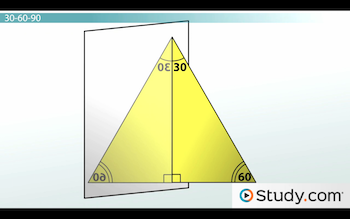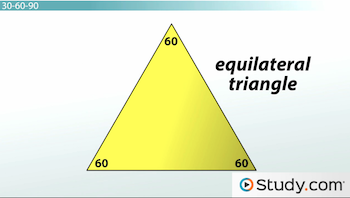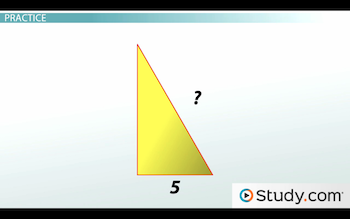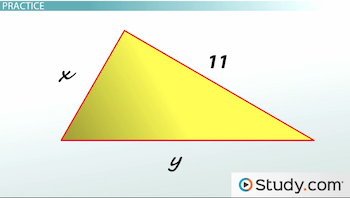# Special Right Triangles: Types and Properties

An error occurred trying to load this video.

Try refreshing the page, or contact customer support.

Coming up next: Law of Sines: Definition and Application

### You're on a roll. Keep up the good work!

Replay
Your next lesson will play in 10 seconds
• 0:06 Special Right Triangles
• 0:42 30-60-90
• 2:26 Practice
• 3:57 45-45-90
• 5:01 Practice
• 5:28 Lesson Summary
Save Save

Want to watch this again later?

Timeline
Autoplay
Autoplay
Speed Speed

#### Recommended Lessons and Courses for You

Lesson Transcript
Instructor: Jeff Calareso

Jeff teaches high school English, math and other subjects. He has a master's degree in writing and literature.

Not all right triangles are the same. In this lesson, we'll look at two special right triangles (30-60-90 and 45-45-90) that have unique properties to help you quickly and easily solve certain triangle problems.

## Special Right Triangles

Triangles are like people. Some are just special in certain ways. For example, maybe you're a great tennis player. You might be the star of your high school's varsity team. That would make you a special tennis player, certainly better than most people at the game. That's like the difference between a right triangle and an oblique triangle. A right triangle probably has a wicked serve.

And then there are special right triangles. These aren't your regional tennis champions. These are your Wimbledon champions. In this lesson, we're going to focus on two right triangle equivalents of tennis royalty.

## 30-60-90

The first special right triangle is the 30-60-90 triangle. I know it sounds like a cell phone plan, but 30-60-90 refers to the triangle's angles.

You know that the side opposite the right angle is the hypotenuse. We call the side opposite the 30-degree angle the short leg. The one opposite the 60-degree angle? That's the long leg. Not too special, but pretty easy to remember.

So, what is special? The ratio of the side lengths. If the hypotenuse is 2, the short leg is 1, and the long leg is the square root of 3.

So, how do you remember which is which? Just think about the logical relative length of the sides. The short leg is the shortest side, so that's your 1. And the long leg is opposite the 60-degree angle, which makes it the middle one, so that's your square root of 3. Finally, the hypotenuse is the longest, and it's opposite the largest angle, so that's your 2.

There's another way to remember this. This triangle is so pretty, let's imagine it looks at itself in the mirror:So, now we have these two 60-degree angles on the bottom, and the 30-degree angle joins its reflected 30-degree angle to become another 60-degree angle. What is this?It's an equilateral triangle, with three equal angles and three equal sides.

So, in this triangle, if one side is 2, then all sides are 2. So, now what would our short leg be? It's half of the bottom side, so it'd be 1.

Of course, you could also use your trigonometry wizardry. Or, if you know two sides, the Pythagorean Theorem. So, that's like four ways to remember the 1:2:sqrt 3 rule.

## Practice

Let's see the 30-60-90 triangle in action. Okay, it's not really going to play tennis or anything, but remembering the ratio can make these problems a breeze.

Here's a 30-60-90 triangle where the short leg is 5:What is the hypotenuse? We know that if the short leg is 1, the hypotenuse is 2. So, whatever we have for the short leg, the hypotenuse is just 2 times that. That means that here, it'll be 10. Problem solved.

What about the long leg? That's going to be 5 times the square root of 3. Sometimes, we'll see 5 root 3 as an answer choice. If so, great! If not, we just need to do the math. The square root of 3 is about 1.73. And 5 times 1.73 is about 9.

Okay, here's a tougher one:In this triangle, the long leg is 11. What are the other two sides? Let's start with the short side, which is x. We can just set up an equation using our ratio. We know it's 1/(root 3), and that equals x/11. Cross multiply to get x(root 3) = 11. Then x = 11/(root 3). And that's going to be about 6.

To unlock this lesson you must be a Study.com Member.

### Register to view this lesson

Are you a student or a teacher?

#### See for yourself why 30 million people use Study.com

##### Become a Study.com member and start learning now.
Back
What teachers are saying about Study.com

### Earning College Credit

Did you know… We have over 200 college courses that prepare you to earn credit by exam that is accepted by over 1,500 colleges and universities. You can test out of the first two years of college and save thousands off your degree. Anyone can earn credit-by-exam regardless of age or education level.